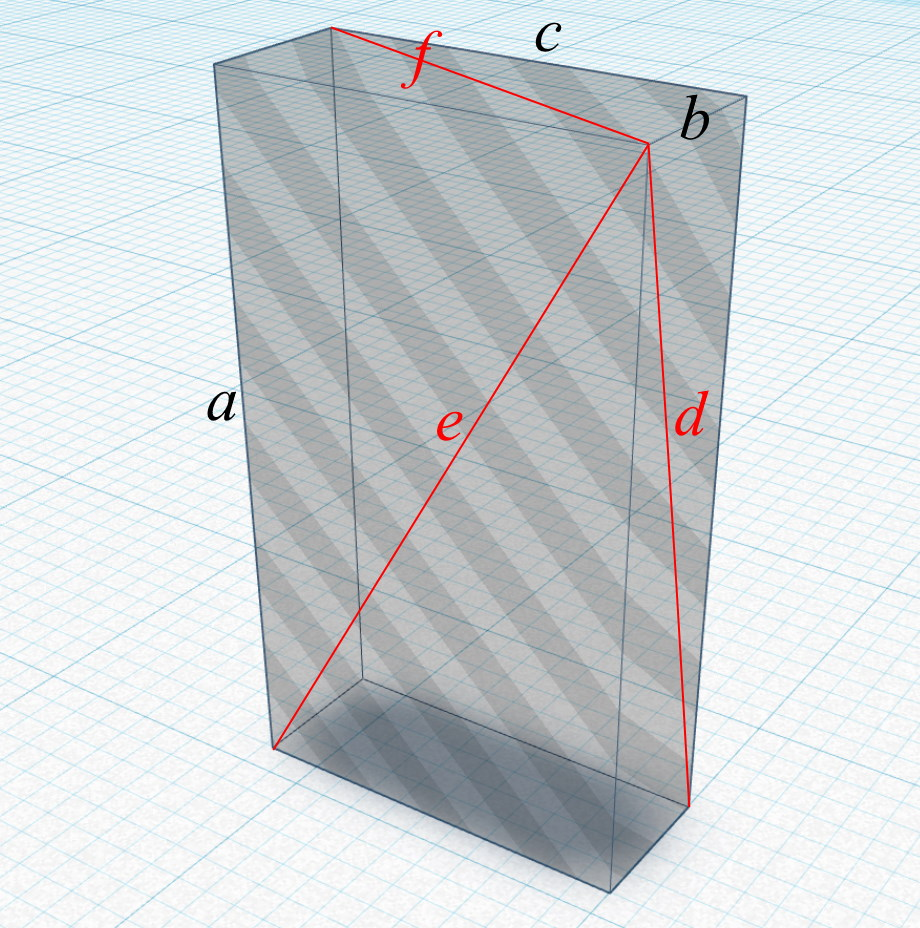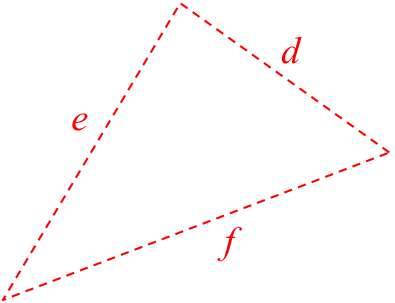# Monoliths and Bricks

There is a classic Sci-Fi novel (and movie), written by Arthur C. Clarke, called 2001: A Space Odyssey.
Prominent in the plot are the appearance of monoliths; cuboids with precise edge ratios of 1 : 4 : 9 (representing the squares of the first three numbers 12 : 22 : 32). The speculation is that these are gestures from an alien intelligence using the universal language of mathematics (Image below).Mathematics as a common language is a popular theme in science fiction movies. In the film Contact (adapted from a novel by Carl Sagan of the same name), signals are picked up from Vega containing a sequence of prime numbers. Prime numbers are fundamental to natural mathematics, and calculation of them is deemed proof of intelligence.
Everyone is a critic, but if I were to rewrite Clarke’s tale, I would have not used the ratio 1 : 4 : 9 but instead 44 : 117 : 240. Why this obscure set of ratios? Well, these ratios are the dimensions of the smallest possible Euler Brick.

## Euler Brick

What is an Euler Brick? An Euler Brick, named after the mathematician Leoonhard Euler, is a cuboid whose edges and face diagonals are all integer lengths.
You are probably familiar with Pythagorean triplets; integer solutions to right angle triangles, such as {3,4,5} and {5,12,13}. These are triangles with integer edges and an integer diagonal (hypotenuse).a2 + b2 = c2
Euler bricks take this a dimension further. All the edges are integers, as are the diagonals of the faces (shown in red below).In algebra they are the solutions to the following Diophantine equations:
a2 + b2 = d2
a2 + c2 = e2
b2 + c2 = f2
Another way to think about this is to think of it like gluing three Pythagorean triplet triangles together to make the common corner.## Examples

Here are some examples of Euler Bricks. The first set of numbers are the edge lengths, the second the face diagonals:
{44,117,240} → {125,244,267}
{85,132,720} → {157,725,732}
{140,480,693} → {500,707,843}
{160,231,792} → {281,808,825}
{187,1020,1584} → {1037,1595,1884}
{195,748,6336} → {773,6339,6380}
{240,252,275} → {348,365,373}
{429,880,2340} → {979,2379,2500}
{495,4888,8160} → {4913,8175,9512}
{528,5796,6325} → {5820,6347,8579}

## Properties

It has been demonstrated that at least two edges of an Euler Brick are divisible by three, at least two are divisible by four, and at least one edge divisible by eleven.